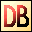DelphiBasicsExpFunction Gives the exponent of a number System unit
 function Exp ( Number : Extended ) : Extended;
Description
The Exp function takes an integer or floating point Number and raises e (2.72) to that power.

This is only used for mathematical processing.

Exp has the opposite effect to Ln - the natural logarithm.
Related commands
 Ln Gives the natural logarithm of a number Log10 Gives the log to base 10 of a numberDownload this web site as a Windows program.